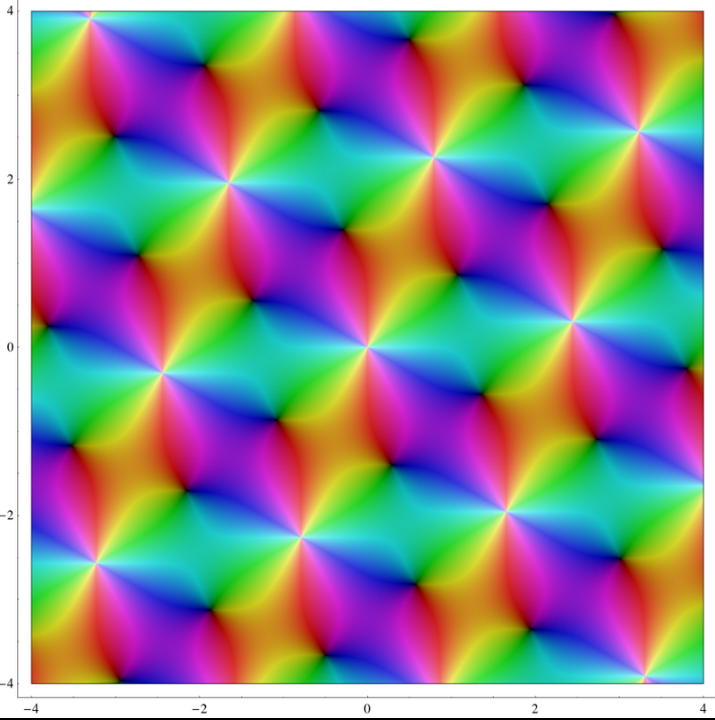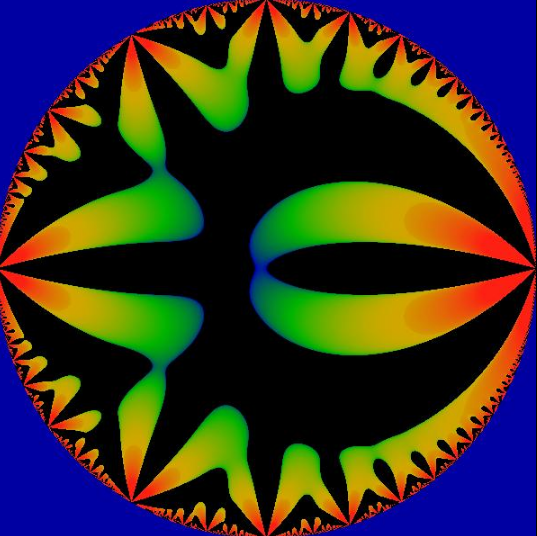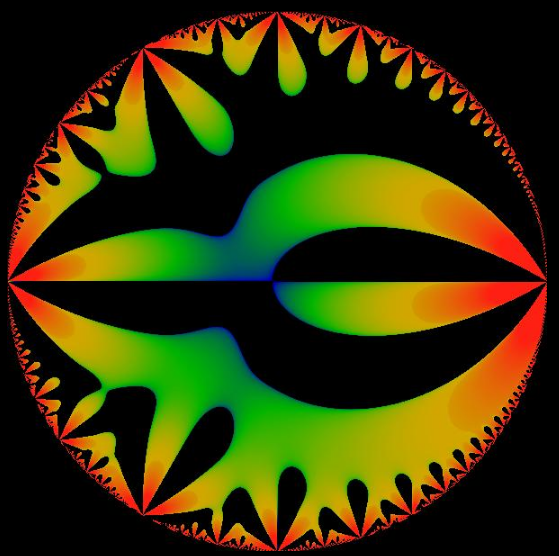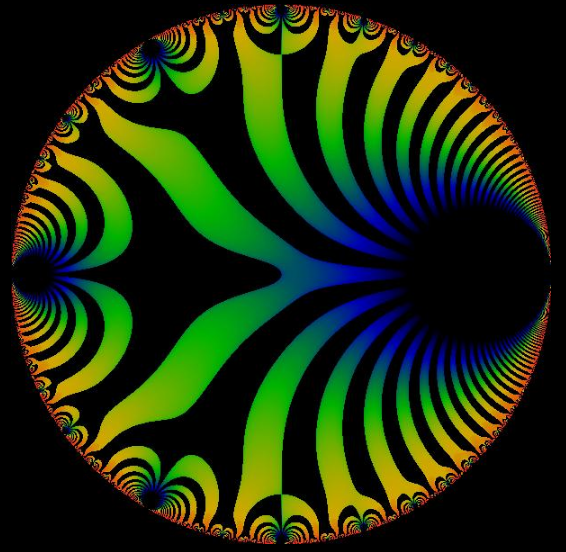Submitted Successfully!
Thank you for your contribution! You can also upload a video entry or images related to this topic.
Ver. Summary Created by Modification Content Size Created at Operation
1 handwiki -- 2540 2022-11-29 01:42:22

Do you have a full video?

# Confirm

Are you sure to Delete?
Cite
Liu, H. Weierstrass's Elliptic Functions. Encyclopedia. Available online: https://encyclopedia.pub/entry/37590 (accessed on 05 December 2023).
Liu H. Weierstrass's Elliptic Functions. Encyclopedia. Available at: https://encyclopedia.pub/entry/37590. Accessed December 05, 2023.
Liu, Handwiki. "Weierstrass's Elliptic Functions" Encyclopedia, https://encyclopedia.pub/entry/37590 (accessed December 05, 2023).
Liu, H.(2022, December 01). Weierstrass's Elliptic Functions. In Encyclopedia. https://encyclopedia.pub/entry/37590
Liu, Handwiki. "Weierstrass's Elliptic Functions." Encyclopedia. Web. 01 December, 2022.
Weierstrass's Elliptic Functions

In mathematics, Weierstrass's elliptic functions are elliptic functions that take a particularly simple form. They are named for Karl Weierstrass. This class of functions are also referred to as p-functions and they are usually denoted by the symbol ℘. They play an important role in theory of elliptic functions. A ℘-function together with its derivative can be used to parameterize elliptic curves and they generate the field of elliptic functions with respect to a given period lattice.

elliptic functions elliptic curves ℘-function

## 1. DefinitionVisualization of the $\displaystyle{ \wp }$-function with invariants $\displaystyle{ g_2=1+i }$ and $\displaystyle{ g_3=2-3i }$ in which white corresponds to a pole, black to a zero. https://handwiki.org/wiki/index.php?curid=1280544

Let $\displaystyle{ \omega_1,\omega_2\in\mathbb{C} }$ be two complex numbers that are linear independent over $\displaystyle{ \mathbb{R} }$ and let $\displaystyle{ \Lambda:=\mathbb{Z}\omega_1+\mathbb{Z}\omega_2:=\{m\omega_1+n\omega_2: m,n\in\mathbb{Z}\} }$ be the lattice generated by those numbers. Then the $\displaystyle{ \wp }$-function is defined as follows:

$\displaystyle{ \weierp(z,\omega_1,\omega_2):=\weierp(z,\Lambda) := \frac{1}{z^2} + \sum_{\lambda\in\Lambda\setminus\{0\}}\left(\frac 1 {(z-\lambda)^2} - \frac 1 {\lambda^2}\right). }$

This series converges locally uniformly absolutely in $\displaystyle{ \mathbb{C}\setminus\Lambda }$. Oftentimes instead of $\displaystyle{ \wp(z,\omega_1,\omega_2) }$ only $\displaystyle{ \wp(z) }$ is written.

The Weierstrass $\displaystyle{ \wp }$-function is constructed exactly in such a way that it has a pole of the order two at each lattice point.

Because the sum $\displaystyle{ \sum_{\lambda\in\Lambda}\frac 1{(z-\lambda)^2} }$ alone would not converge it is necessary to add the term $\displaystyle{ -\frac 1 {\lambda^2} }$.

It is common to use $\displaystyle{ 1 }$ and $\displaystyle{ \tau\in\mathbb{H}:=\{z\in\mathbb{C}:\operatorname{Im}(z)\gt 0\} }$ as generators of the lattice. Multiplying by $\displaystyle{ \frac 1{\omega_1} }$ maps the lattice $\displaystyle{ \mathbb{Z}\omega_1+\mathbb{Z}\omega_2 }$ isomorphically onto the lattice $\displaystyle{ \mathbb{Z}+\mathbb{Z}\tau }$ with $\displaystyle{ \tau=\frac{\omega_2}{\omega_1} }$. By possibly substituting $\displaystyle{ \tau }$ by $\displaystyle{ -\tau }$ it can be assumed that $\displaystyle{ \tau\in\mathbb{H} }$. One sets $\displaystyle{ \wp(z,\tau) := \wp(z, 1,\tau) }$.

## 2. Motivation

A cubic of the form $\displaystyle{ C_{g_2,g_3}^\mathbb{C}=\{(x,y)\in\mathbb{C}^2:y^2=4x^3-g_2x+g_3\} }$, where $\displaystyle{ g_2,g_3\in\mathbb{C} }$ are complex numbers with $\displaystyle{ g_2^3-27g_3^2\neq0 }$, can not be rationally parameterized. Yet one still wants to find a way to parameterize it.

For the quadric $\displaystyle{ K=\{(x,y)\in\mathbb{R}^2:x^2+y^2=1\} }$, the unit circle, there exists a (non-rational) parameterization using the sine function and its derivative the cosine function:

$\displaystyle{ \psi:\mathbb{R}/2\pi\mathbb{Z}\to K, \quad t\mapsto(\sin(t),\cos(t)) }$.

Because of the periodicity of the sine and cosine $\displaystyle{ \mathbb{R}/2\pi\mathbb{Z} }$ is chosen to be the domain, so the function is bijective.

In a similar way one can get a parameterization of $\displaystyle{ C_{g_2,g_3}^\mathbb{C} }$ by means of the doubly periodic $\displaystyle{ \wp }$-function (see in the section "Relation to ellitpic curves"). This parameterization has the domain $\displaystyle{ \mathbb{C}/\Lambda }$, which is topologically equivalent to a torus.

There is another analogy to the trigonometric functions. Consider the integral function

$\displaystyle{ a(x)=\int_0^x\frac{dy}{\sqrt{(1-y^2)}} }$.

It can be simplified by substituting $\displaystyle{ y=\sin(t) }$ and $\displaystyle{ s=\arcsin(x) }$:

$\displaystyle{ a(x)=\int_0^sdt=s=\arcsin(x) }$.

That means $\displaystyle{ a^{-1}(x)=\sin(x) }$. So the sine function is an inverse function of an integral function.

Elliptic functions are also inverse functions of integral functions, namely of elliptic integrals. In particular the $\displaystyle{ \wp }$-function is obtained in the following way:

Let

$\displaystyle{ u(z)=-\int_z^\infin\frac{ds}{\sqrt{4s^3-g_2s-g_3}} }$.

Then $\displaystyle{ u^{-1} }$ can be extended to the complex plane and this extension equals the $\displaystyle{ \wp }$-function.

## 3. Properties

• ℘ is an even function. That means $\displaystyle{ \wp(z)=\wp(-z) }$ for all $\displaystyle{ z \in \mathbb{C} \setminus \Lambda }$, which can be seen in the following way:
$\displaystyle{ \weierp(-z)=\frac{1}{(-z)^2}+\sum_{\lambda\in\Lambda\setminus\{0\}}\left(\frac1{(-z-\lambda)^2}-\frac1{\lambda^2}\right)=\frac{1}{z^2}+\sum_{\lambda\in\Lambda\setminus\{0\}}\left(\frac1{(z+\lambda)^2}-\frac1{\lambda^2}\right)=\frac{1}{z^2}+\sum_{\lambda\in\Lambda\setminus\{0\}}\left(\frac1{(z-\lambda)^2}-\frac1{\lambda^2}\right)=\wp(z) }$

The second last equality holds because $\displaystyle{ \{-\lambda:\lambda \in \Lambda\}=\Lambda }$. Since the sum converges absolutely this rearrangement does not change the limit.

• ℘ is meromorphic and its derivative is
$\displaystyle{ \wp'(z)=-2\sum_{\lambda \in \Lambda}\frac1{(z-\lambda)^3} }$.
• $\displaystyle{ \wp }$ and $\displaystyle{ \wp' }$ are doubly periodic with the periods $\displaystyle{ \omega_1 }$und $\displaystyle{ \omega_2 }$. This means:
$\displaystyle{ \wp(z+\omega_1)=\wp(z)=\wp(z+\omega_2) }$ and $\displaystyle{ \wp'(z+\omega_1)=\wp'(z)=\wp'(z+\omega_2) }$.

It follows that $\displaystyle{ \wp(z+\lambda)=\wp(z) }$ and $\displaystyle{ \wp'(z+\lambda)=\wp'(z) }$ for all $\displaystyle{ \lambda \in \Lambda }$. Functions which are meromorphic and doubly periodic are also called elliptic functions.

## 4. Laurent Expansion

Let $\displaystyle{ r:=\min\{{|\lambda}|:0\neq\lambda\in\Lambda\} }$. Then for $\displaystyle{ 0\lt |z|\lt r }$ the $\displaystyle{ \wp }$-function has the following Laurent expansion

$\displaystyle{ \wp(z)=\frac1{z^2}+\sum_{n=1}^\infin (2n+1)G_{2n+2}z^{2n} }$

where

$\displaystyle{ G_n=\sum_{0\neq\lambda\in\Lambda}\lambda^{-n} }$ for $\displaystyle{ n\geq3 }$ are so called Eisenstein series.

## 5. Differential Equation

Set $\displaystyle{ g_2=60G_4 }$ and $\displaystyle{ g_3=140G_6 }$. Then the $\displaystyle{ \wp }$-function satisfies the differential equation

$\displaystyle{ \wp'^2(z) = 4\wp ^3(z)-g_2\wp(z)-g_3 }$.

This relation can be verified by forming a linear combination of powers of $\displaystyle{ \wp }$ and $\displaystyle{ \wp' }$ to eliminate the pole at $\displaystyle{ z=0 }$. This yields an entire elliptic function that has to be constant by Liouville's theorem .

## 6. InvariantsThe real part of the invariant g3 as a function of the nome q on the unit disk. https://handwiki.org/wiki/index.php?curid=1389931The imaginary part of the invariant g3 as a function of the nome q on the unit disk. https://handwiki.org/wiki/index.php?curid=1178918

The coefficients of the above differential equation g2 and g3 are known as the invariants. Because they depent on the lattice $\displaystyle{ \Lambda }$ they can be viewed as functions in $\displaystyle{ \omega_1 }$and $\displaystyle{ \omega_2 }$.

The series expansion suggests that g2 and g3 are homogeneous functions of degree −4 and −6. That is

$\displaystyle{ g_2(\lambda \omega_1, \lambda \omega_2) = \lambda^{-4} g_2(\omega_1, \omega_2) }$
$\displaystyle{ g_3(\lambda \omega_1, \lambda \omega_2) = \lambda^{-6} g_3(\omega_1, \omega_2) }$ for $\displaystyle{ \lambda\neq0 }$.

If $\displaystyle{ \omega_1 }$and $\displaystyle{ \omega_2 }$ are chosen in such a way that $\displaystyle{ \operatorname{Im}\left( \frac{\omega_2}{\omega_1} \right)\gt 0 }$ g2 and g3 can be interpreted as functions on the upper half-plane $\displaystyle{ \mathbb{H}:=\{z\in\mathbb{C}:\operatorname{Im}(z)\gt 0\} }$.

Let $\displaystyle{ \tau=\frac{\omega_2}{\omega_1} }$. One has:

$\displaystyle{ g_2(1,\tau)=\omega_1^4g_2(\omega_1,\omega_2) }$,
$\displaystyle{ g_3(1,\tau)=\omega_1^6 g_3(\omega_1,\omega_2) }$.

That means g2 and g3 are only scaled by doing this. Set

$\displaystyle{ g_2(\tau):=g_2(1,\tau) }$, $\displaystyle{ g_3(\tau):=g_3(1,\tau) }$.

As functions of $\displaystyle{ \tau\in\mathbb{H} }$ $\displaystyle{ g_2,g_3 }$ are so called modular forms.

The Fourier series for $\displaystyle{ g_2 }$ and $\displaystyle{ g_3 }$ are given as follows:

$\displaystyle{ g_2(\tau)=\frac{4}{3}\pi^4 \left[ 1+ 240\sum_{k=1}^\infty \sigma_3(k) q^{2k} \right] }$
$\displaystyle{ g_3(\tau)=\frac{8}{27}\pi^6 \left[ 1- 504\sum_{k=1}^\infty \sigma_5(k) q^{2k} \right] }$

where $\displaystyle{ \sigma_a(k):=\sum_{d\mid{k}}d^\alpha }$ is the divisor function and $\displaystyle{ q:=\exp(i\pi\tau) }$.

## 7. Modular DiscriminantThe real part of the discriminant as a function of the nome q on the unit disk.

The modular discriminant Δ is defined as the discriminant of the polynomial at right-hand side of the above differential equation:

$\displaystyle{ \Delta=g_2^3-27g_3^2. \, }$

The discriminant is a modular form of weight 12. That is, under the action of the modular group, it transforms as

$\displaystyle{ \Delta \left( \frac {a\tau+b} {c\tau+d}\right) = \left(c\tau+d\right)^{12} \Delta(\tau) }$

where $\displaystyle{ a,b,d,c\in\mathbb{Z} }$ with ad − bc = 1.

Note that $\displaystyle{ \Delta=(2\pi)^{12}\eta^{24} }$ where $\displaystyle{ \eta }$ is the Dedekind eta function.

For the Fourier coefficients of $\displaystyle{ \Delta }$, see Ramanujan tau function.

## 8. The Constants e1, e2 and e3

$\displaystyle{ e_1 }$, $\displaystyle{ e_2 }$ and $\displaystyle{ e_3 }$ are usually used to denote the values of the $\displaystyle{ \wp }$-function at the half-periods.

$\displaystyle{ e_1\equiv\wp\left(\frac{\omega_1}{2}\right) }$
$\displaystyle{ e_2\equiv\wp\left(\frac{\omega_2}{2}\right) }$
$\displaystyle{ e_3\equiv\wp\left(\frac{\omega_1+\omega_2}{2}\right) }$

They are pairwise distinct and only depend on the lattice $\displaystyle{ \Lambda }$ and not on its generators.

$\displaystyle{ e_1 }$, $\displaystyle{ e_2 }$ and $\displaystyle{ e_3 }$ are the roots of the cubic polynomial $\displaystyle{ 4\wp(z)^3-g_2\wp(z)-g_3 }$ and are related by the equation:

$\displaystyle{ e_1+e_2+e_3=0 }$.

Because those roots are distinct the discriminant $\displaystyle{ \Delta }$ does not vanish on the upper half plane. Now we can rewrite the differential equation:

$\displaystyle{ \wp'^2(z)=4(\wp(z)-e_1)(\wp(z)-e_2)(\wp(z)-e_3) }$.

That means the half-periods are zeros of $\displaystyle{ \wp' }$.

The invariants $\displaystyle{ g_2 }$ and $\displaystyle{ g_3 }$ can be expressed in terms of these constants in the following way:

$\displaystyle{ g_2=-4(e_1e_2+e_1e_3+e_2e_3) }$
$\displaystyle{ g_3=4e_1e_2e_3 }$

## 9. Relation to Elliptic Curves

Consider the projective cubic curve

$\displaystyle{ \bar C_{g_2,g_3}^\mathbb{C}=\{(x,y)\in\mathbb{C}^2:y^2=4x^3-g_2x+g_3\}\cup\{\infin\}\subset\mathbb{P}_\mathbb{C}^2 }$.

For this cubic, also called Weierstrass cubic, there exists no rational parameterization, if $\displaystyle{ \Delta\neq0 }$. In this case it is also called an elliptic curve. Nevertheless there is a parameterization that uses the $\displaystyle{ \wp }$-function and its derivative $\displaystyle{ \wp' }$:

$\displaystyle{ \varphi: \mathbb{C}/\Lambda\to\bar C_{g_2,g_3}^\mathbb{C}, \quad \bar{z}\mapsto \begin{cases} (\wp(z),\wp'(z),1) & \bar{z}\neq0\\ \infin \quad &\bar{z}=0 \end{cases} }$

Now the map $\displaystyle{ \varphi }$ is bijective and parameterizes the elliptic curve $\displaystyle{ \bar C_{g_2,g_3}^\mathbb{C} }$.

$\displaystyle{ \mathbb{C}/\Lambda }$ is an abelian group and a topological space, equipped with the quotient topology.

It can be shown that every Weierstrass cubic is given in such a way. That is to say that for every pair $\displaystyle{ g_2,g_3\in\mathbb{C} }$ with $\displaystyle{ \Delta=g_2^3-27g_3^2\neq0 }$ there exists a lattice $\displaystyle{ \mathbb{Z}\omega_1+\mathbb{Z}\omega_2 }$, such that

$\displaystyle{ g_2=g_2(\omega_1,\omega_2) }$ and $\displaystyle{ g_3=g_3(\omega_1,\omega_2) }$.

The statement that elliptic curves over $\displaystyle{ \mathbb{Q} }$ can be parameterized over $\displaystyle{ \mathbb{Q} }$, is known as the modularity theorem. This is an important theorem in number theory. It was part of Andrew Wiles' proof (1995) of Fermat's Last Theorem.

Let $\displaystyle{ z,w\in\mathbb{C} }$, so that $\displaystyle{ z,w,z+w,z-w\notin\Lambda }$. Then one has:

$\displaystyle{ \wp(z+w)=\frac1{4} \left[\frac{\wp'(z)-\wp'(w)}{\wp(z)-\wp(w)}\right]^2-\wp(z)-\wp(w) }$.

As well as the duplication formula:

$\displaystyle{ \wp(2z)=\frac1{4}\left[\frac{\wp''(z)}{\wp'(z)}\right]^2-2\wp(z) }$.

These formulas also have a geometric interpretation, if one looks at the elliptic curve $\displaystyle{ \bar C_{g_2,g_3}^\mathbb{C} }$ together with the mapping $\displaystyle{ {\varphi}:\mathbb{C}/\Lambda\to\bar C_{g_2,g_3}^\mathbb{C} }$ as in the previous section.

The group structure of $\displaystyle{ (\mathbb{C}/\Lambda,+) }$ translates to the curve $\displaystyle{ \bar C_{g_2,g_3}^\mathbb{C} }$and can be geometrically interpreted there:

The sum of three pairwise different points $\displaystyle{ a,b,c\in\bar C_{g_2,g_3}^\mathbb{C} }$is zero if and only if they lie on the same line in $\displaystyle{ \mathbb{P}_\mathbb{C}^2 }$.

This is equivalent to:

$\displaystyle{ \det\left(\begin{array}{rrr} 1&\wp(u+v)&-\wp'(u+v)\\ 1&\wp(v)&\wp'(v)\\ 1&\wp(u)&\wp'(u)\\ \end{array}\right) =0 }$,

where $\displaystyle{ \wp(u)=a }$, $\displaystyle{ \wp(v)=b }$ and $\displaystyle{ u,v\notin\Lambda }$.

## 11. Relation to Jacobi's Elliptic Functions

For numerical work, it is often convenient to calculate the Weierstrass elliptic function in terms of Jacobi's elliptic functions.

The basic relations are:

$\displaystyle{ \wp(z) = e_3 + \frac{e_1 - e_3}{\operatorname{sn}^2 w} = e_2 + ( e_1 - e_3 ) \frac{\operatorname{dn}^2 w}{\operatorname{sn}^2 w} = e_1 + ( e_1 - e_3 ) \frac{\operatorname{cn}^2 w}{\operatorname{sn}^2 w} }$

where $\displaystyle{ e_1,e_2 }$and $\displaystyle{ e_3 }$ are the three roots described above and where the modulus k of the Jacobi functions equals

$\displaystyle{ k =\sqrt{\frac{e_2 - e_3}{e_1 - e_3}} }$

and their argument w equals

$\displaystyle{ w =z \sqrt{e_1 - e_3}. }$

## 12. Typography

The Weierstrass's elliptic function is usually written with a rather special, lower case script letter ℘.

In computing, the letter ℘ is available as \wp in TeX. In Unicode the code point is U+2118SCRIPT CAPITAL P (HTML &#8472; · &weierp;), with the more correct alias weierstrass elliptic function. In HTML, it can be escaped as &weierp;.

Character information
Preview Template:Charmap/showcharTemplate:Charmap/showcharTemplate:Charmap/showcharTemplate:Charmap/showchar
Unicode name SCRIPT CAPITAL P / WEIERSTRASS ELLIPTIC FUNCTION
Encodings decimal hex
Unicode 8472 0 0 0 U+2118
UTF-8 226 132 152 0 0 0 E2 84 98 00 00 00
Numeric character reference &#8472;&#0;&#0;&#0; &#x2118;&#x00;&#x00;&#x00;
Named character reference &weierp;

### References

1. Apostol, Tom M. (1976). Modular functions and Dirichlet series in number theory. New York: Springer-Verlag. pp. 9. ISBN 0-387-90185-X. OCLC 2121639. https://www.worldcat.org/oclc/2121639.
2. Hulek, Klaus. (2012) (in German), Elementare Algebraische Geometrie : Grundlegende Begriffe und Techniken mit zahlreichen Beispielen und Anwendungen (2., überarb. u. erw. Aufl. 2012 ed.), Wiesbaden: Vieweg+Teubner Verlag, p. 8, ISBN 978-3-8348-2348-9
3. Rolf Busam (2006) (in German), Funktionentheorie 1 (4., korr. und erw. Aufl ed.), Berlin: Springer, p. 259, ISBN 978-3-540-32058-6
4. Jeremy Gray (2015) (in German), Real and the complex : a history of analysis in the 19th century, Cham, p. 71, ISBN 978-3-319-23715-2
5. Rolf Busam (2006) (in German), Funktionentheorie 1 (4., korr. und erw. Aufl ed.), Berlin: Springer, p. 294, ISBN 978-3-540-32058-6
6. Apostol, Tom M. (1976) (in German), Modular functions and Dirichlet series in number theory, New York: Springer-Verlag, p. 11, ISBN 0-387-90185-X
7. Apostol, Tom M. (1976). Modular functions and Dirichlet series in number theory. New York: Springer-Verlag. pp. 14. ISBN 0-387-90185-X. OCLC 2121639. https://www.worldcat.org/oclc/2121639.
8. Apostol, Tom M. (1976) (in German), Modular functions and Dirichlet series in number theory, New York: Springer-Verlag, p. 14, ISBN 0-387-90185-X
9. Apostol, Tom M. (1990). Modular functions and Dirichlet series in number theory (2nd ed.). New York: Springer-Verlag. pp. 20. ISBN 0-387-97127-0. OCLC 20262861. https://www.worldcat.org/oclc/20262861.
10. Apostol, Tom M. (1976). Modular functions and Dirichlet series in number theory. New York: Springer-Verlag. pp. 50. ISBN 0-387-90185-X. OCLC 2121639. https://www.worldcat.org/oclc/2121639.
11. Chandrasekharan, K. (Komaravolu), 1920- (1985). Elliptic functions. Berlin: Springer-Verlag. pp. 122. ISBN 0-387-15295-4. OCLC 12053023. https://www.worldcat.org/oclc/12053023.
12. Rolf Busam (2006) (in German), Funktionentheorie 1 (4., korr. und erw. Aufl ed.), Berlin: Springer, p. 270, ISBN 978-3-540-32058-6
13. Tom M. Apostol (1976) (in German), Modular functions and Dirichlet series in number theory, New York: Springer-Verlag, p. 13, ISBN 0-387-90185-X
14. K. Chandrasekharan (1985) (in German), Elliptic functions, Berlin: Springer-Verlag, p. 33, ISBN 0-387-15295-4
15. Hulek, Klaus. (2012) (in German), Elementare Algebraische Geometrie : Grundlegende Begriffe und Techniken mit zahlreichen Beispielen und Anwendungen (2., überarb. u. erw. Aufl. 2012 ed.), Wiesbaden: Vieweg+Teubner Verlag, p. 12, ISBN 978-3-8348-2348-9
16. Hulek, Klaus. (2012) (in German), Elementare Algebraische Geometrie : Grundlegende Begriffe und Techniken mit zahlreichen Beispielen und Anwendungen (2., überarb. u. erw. Aufl. 2012 ed.), Wiesbaden: Vieweg+Teubner Verlag, p. 111, ISBN 978-3-8348-2348-9
17. Rolf Busam (2006) (in German), Funktionentheorie 1 (4., korr. und erw. Aufl ed.), Berlin: Springer, p. 286, ISBN 978-3-540-32058-6
18. Rolf Busam (2006) (in German), Funktionentheorie 1 (4., korr. und erw. Aufl ed.), Berlin: Springer, p. 287, ISBN 978-3-540-32058-6
19. Rolf Busam (2006) (in German), Funktionentheorie 1 (4., korr. und erw. Aufl ed.), Berlin: Springer, p. 288, ISBN 978-3-540-32058-6
20. Korn GA, Korn TM (1961). Mathematical Handbook for Scientists and Engineers. New York: McGraw–Hill. pp. 721.
21. This symbol was used already at least in 1890. The first edition of A Course of Modern Analysis by E. T. Whittaker in 1902 also used it.
22. The Unicode Consortium has acknowledged two problems with the letter's name: the letter is in fact lowercase, and it is not a "script" class letter, like U+1D4C5 ? MATHEMATICAL SCRIPT SMALL P, but the letter for Weierstrass's elliptic function. Unicode added the alias as a correction.
More
Information
Subjects:
Contributor MDPI registered users' name will be linked to their SciProfiles pages. To register with us, please refer to https://encyclopedia.pub/register :
View Times: 612
Entry Collection:
Revision: 1 time (View History)
Update Date: 01 Dec 2022
1000/1000